2022-02-18
###### Hyperparameter optimization techniques in machine learning with Python code
2022-02-22

Linear Regression is commonly the first machine learning problem that people are interested in in the area of study. For those who have some experience with ML, sometimes this technique is boring, due to its simplicity (and, of course, limitations). I had this opinion until I decided to revisit the math behind ML models to construct a solid base of knowledge and then faced the Linear Regression technique again. To my surprise, this technique has a solid and interesting math formulation that most times we don’t see. In this post, I want to detail such ideas and I hope it will help you to understand the whole formulation instead of just applying it.

First of all, for the sake of conciseness, I will not describe the problem: I consider that the reader already has a basic understanding of such a technique and its application as a squared loss optimization problem solved by gradient descent. Actually, in this post, I will describe the mathematical formulation of Linear Regression from the perspective of Maximum Likelihood Estimation (MLE), Maximum a Posteriori (MAP), and Bayesian Linear Regression (which means we will not use gradient descent — but closed analytical solutions that solve linear regression problems).

## Problem Formulation

Given the supervised learning problem of fitting the dataset consisting of pairs (x,y), we consider a regression problem with the likelihood function

It means that we have the following functional relationship between the data:

where epsilon is a gaussian variable with zero mean and variance σ², an i.i.d. measurement noise. The objective is to find a predictor function that is similar to this true data generator function. In the Linear Regression formulation, as a parametric model, we consider that such function is a linear combination of parameters and the data vector:

It is important to mention that we consider the bias parameter as an element of the θ parameter (and, hence, we concatenate a “1” at the end of the data vector). This formulation facilitates the math description. Therefore, our linear regression model is given by:

where such probability density function is called likelihood, and the whole uncertainty of this model is due to the observation noise epsilon. Based on this formulation, it is clear that our mission is to find the best parameter vector θ that spans the best predictor function. We will derive linear regression equations by the view of parameter estimation and using Bayesian statistics.

## Parameter Estimation

Considering our training set D as composed of inputs X and targets Y, the likelihood of our training set can be mathematically described as:

By formulation, our data is i.i.d. In a probabilistic view, it means that we can factorize the equation above as the production of each data sample:

From the parameter estimation viewpoint, the training process aims to estimate a single point value for each parameter using the knowledge of our training set. In this post we will describe the parameter estimation by two techniques: Maximum Likelihood Estimation (MLE) and Maximum a Posteriori (MAP).

## Maximum Likelihood Estimation

In MLE, we find the parameters θₘₗₑ by maximizing the likelihood:

Intuitively, maximizing likelihood means that we will vary the parameter vector in a way to “enlarge” the probability of the predictor function outputs the observation y for each corresponding input vector — which is just what we want.

Instead of using the production form of the likelihood, we apply a log transformation for two great reasons: to facilitate derivative calculations and avoid underflows due to several products of small decimal values. As the log function is a strictly monotonic increasing function, we do not change the optimization critical points. Additionally, we are familiarized with minimizing the cost function. So let’s convert it to a minimization problem as well. Therefore:

This is the so important idea of “minimizing negative log-likelihood” in machine learning problems! More than that: if we consider our problem formulation of likelihood as a gaussian variable, we can derive:

Yes! In the context of linear regression, if we formulate our likelihood as a gaussian distribution, maximizing such function is similar to minimizing the squared error! Very nice, isn’t it? This is also the reason why we use MSE loss in the gradient descent.

We can find an analytic solution for this problem. This loss function is quadratic in θ, so we can conclude there is only one global optimum. Therefore, we can find the global optimum by computing its gradient and set to zero:

It is worth mentioning that the zero gradient is necessary and enough condition for this critical point to be a global minimum due to Hessian analysis. In fact, we have:

As is a full-rank matrix, then the Hessian above is a positive-definite matrix.

Before we dive into the code, it is important to mention that linear regression means that there is a linear relationship between the parameters and the inputs. Hence, a polynomial regression can also be considered a linear regression, where:

and:

There is an implementation of this analytic solution for Linear Regression in ml-room:

from predictor import Predictor
import numpy as np

class LinearRegressionMLE(Predictor):
def __init__(self):
self.weights = None

def train(self, train_x, train_y):
bias = np.ones((train_x.shape, 1))
X = np.concatenate((train_x, bias), axis = 1)
self.weights = np.linalg.inv(X.T.dot(X)).dot(X.T).dot(train_y)

def predict(self, test_x):
bias = np.ones((test_x.shape, 1))
X = np.concatenate((test_x, bias), axis = 1)
return X.dot(self.weights)


I also coded a gradient descent linear regression to compare the results of each regression. You may see all the test cases in the main.py file.

Comparing a simple case where we would like to fit a linear function, we have for the case of 1000 epochs of gradient descent the following result:

Epoch: 999Cost: 24.748796723940572Gradients (W, b): 0.007573801056179228, -0.03123069273204225Weights: [[-4.10382742]], [[7.29646987]]train: 0.41993188858 seconds.predict: 1.19209289551e-05 seconds.Squared Mean Error: 24.748685809688233

On the other side, in the case of the MLE analytic solution:

Test Linear Regression MLE: train: 0.000187158584595 seconds.predict: 2.40802764893e-05 seconds.Squared Mean Error: 27.063798011530853

Well, in both cases the error is similar (this variation is probably due to the high noise). However, the analytic solution is much faster during training! Of course, we can tune better the hyperparameters of gradient descent optimization, but it will not surpass the performance of the MLE solution during training.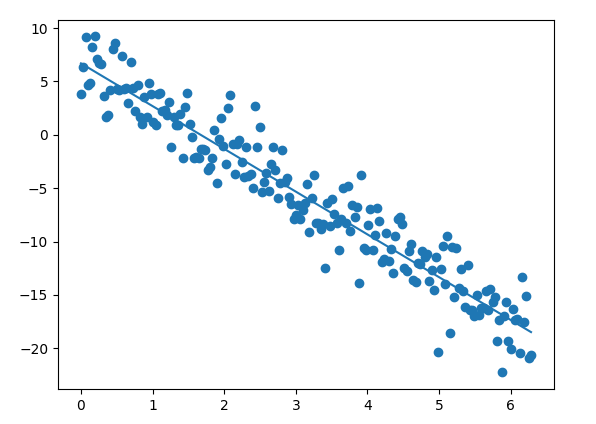Linear Regression MLE solution for y = -4*x + 7 + noise*2.0

We could also estimate the noise variance of the dataset analytically. We just need to consider the σ as part of the model and derivate w.r.t it and find the global maximum again…

Finally, there is a geometric view for the Maximum Likelihood Estimation. If you analyze the closed form of MLE for Linear Regression, we have:

We are looking for the solution of y = Xθ, and the reconstruction of training targets is given by:

This formula means an orthogonal projection of onto the one-dimensional subspace spanned by X. Actually,

is the projection matrix. Therefore, the MLE provides the geometrically optimal solution by finding the vectors in the subspace spanned by that has the minimum squared distance to the corresponding targets — this is the orthogonal projection. The figure below illustrates this idea.

## Maximum a Posteriori Estimation

MLE is a great parameter estimation technique for linear regression problems. However, it is prone to overfitting. This problem is clear when we talk about polynomial regression. If we have enough parameters (i.e, enough capacity of representation by our prediction function), the MLE will generate parameters with a high magnitude which will span a function that will oscillate wildly and pass through each data point, generating a poor representation of our data generator function. In ML, we don’t just need to minimize the error, but also achieve a good generalization.

In order to mitigate the effect of huge parameter values, we can place a prior distribution p(θ) on the parameters, which will encode the range of possible values. From Bayes’ theorem:

In MAP estimation, instead of maximizing the likelihood, we maximize the posterior function p(θ ∣ X, Y). This function encodes the information from the likelihood and the prior distribution. In order to find the solution, we follow the same log transformation:

We consider const everything independent of the parameters. Again:

We also consider the prior a conjugate gaussian distribution, which means that the posterior distribution will also be a gaussian (check about Conjugacy distributions). Therefore:

Calculating the gradient and setting it to zero (the conditions of positive definiteness remain the same):

Considering MLE, we can see that the difference between both estimations is the term that relates the variance of observation noise and the variance of our prior distribution.

Now, focus on the “loss” function of MAP. In comparison to the MLE, we just added a term:

We basically added a regularization term! Hence: when we consider an L2 regularization in a loss function, we are placing a prior distribution to our parameters! This distribution defines where the parameters are, which avoids huge parameters by shrinking them. It is also possible to derive that the λ term, commonly used in linear regression, is relative to the variances from the observation noise and prior distributions.

You can see the implementation of MAP estimation below.


from predictor import Predictor
import numpy as np

class LinearRegressionMAP(Predictor):
def __init__(self):
self.weights = None

def train(self, train_x, train_y, lambd):
bias = np.ones((train_x.shape, 1))
X = np.concatenate((train_x, bias), axis = 1)
self.weights = np.linalg.inv(X.T.dot(X) + lambd*np.eye(X.shape)).dot(X.T).dot(train_y)

def predict(self, test_x):
bias = np.ones((test_x.shape, 1))
X = np.concatenate((test_x, bias), axis = 1)
return X.dot(self.weights)


As a test scenario, I tried to fit a sinusoidal function by a polynomial of degree 9. As you can see, the MLE has a poor representation, because it has high variance (you can check to see the weird shape trying to pass through the data points). On the other side, the MAP estimation has a shape more similar to the trigonometric function — that’s the regularization acting!

Here we finalize the parameter estimation section. But before finalizing our Linear Regression post, let’s derive another solution by a Bayesian viewpoint.

## Bayesian Linear Regression

In Bayesian Linear Regression, instead of computing a point estimation of the parameter vector, we consider such parameters as random variables and compute a full posterior over them, which means an average over all plausible parameter settings.

We consider the model:

where ϕ(x) is the generalized input vector.

We will derive the posterior distribution directly (instead of talking about prior inference) and look for this model in practice. The posterior over the parameters using Bayes’ Theorem is:

From our model, we already have the prior and likelihood distributions. The distribution p(Y ∣ X) is called marginal likelihood and is calculated by:

This distribution is independent of the parameters and ensures the posterior is normalized. We can think of the marginal likelihood as the likelihood averaged over all possible parameter settings, accordingly to the prior distribution.

Using those three distributions and the Bayes’ Theorem we found that the posterior distribution is:

I preferred not to derive mathematically this result here for the sake of conciseness. But the process is about applying the log transformation to the Bayes’ expression, doing some matrix algebra, and then identifying the mean and covariance matrix. Furthermore, we exploit the fact that the marginal likelihood is independent of θ and, therefore, its term is just a constant after log transformation. For detailed derivation, check here.

Now that we have the posterior distribution, we need to compute the posterior prediction:

This expression will give us the distribution over the observation space — the uncertainty over the prediction. Basically, is the region where the prediction lies. To calculate such distribution, we integrate over the posterior distribution:

The derivation of this formula follows the idea of conjugacy of Gaussians and the marginalization property of Gaussian distributions.

Here is the code for this analytical solution:

from predictor import Predictor
import numpy as np

class BayesianLinearRegression(Predictor):
def __init__(self):
self.m_n = None
self.S_n = None
self.sigma = None

def train(self, train_x, train_y, sigma, m0, s0):
bias = np.ones((train_x.shape, 1))
X = np.concatenate((train_x, bias), axis = 1)
inv_s0 = np.linalg.inv(s0)
self.S_n = np.linalg.inv(inv_s0 + (1/(sigma**2))*X.T.dot(X))
self.sigma = sigma
self.m_n = self.S_n.dot(inv_s0.dot(m0) + (1/(sigma**2))*X.T.dot(train_y))

def predict(self, test_x):
bias = np.ones((test_x.shape, 1))
X = np.concatenate((test_x, bias), axis = 1)
mu = X.dot(self.m_n).flatten()
var = np.array(map(lambda x: x.dot(self.S_n).dot(x.T)+ self.sigma**2, X))
return np.random.normal(mu, var), mu, var



As a test case, I use the same case of polynomial regression previously analyzed. As result, we have:

In this plot, the scatter plot refers to the input data points. The blue line is the mean of the distribution estimated for each data point. The blue shaded area forms the 95% confidence bounds. As we can see, our result is not just a single line of predictions (resulting from point estimates of the parameters vector), but actually, it is a “region” estimation (resulting from distributions for each parameter).

## Summary

In this, post, I explained some of the most important statistical concepts for ML theory using Linear Regression. This technique is great for this explanation because of its closed analytical form when using gaussian distributions. In other cases, we need to solve harder optimization problems, and for that, we commonly use gradient descent optimization.

### Maximum Likelihood Estimation (MLE)

MLE is the most common way in machine learning to estimate the model parameters that fit into the given data, especially when the model is getting complex such as deep learning. MLE falls into the frequentist view, which simply gives a single estimate that maximizes the probability of a given observation.

Formally, we can denote MLE as: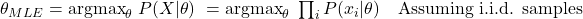where θ is the parameters and X is the observation. Since calculating the product of probabilities (between 0 to 1) is not numerically stable in computers, we add the log term to make it computable: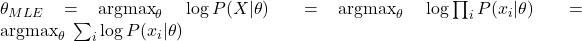Therefore, we usually say we optimize the “log likelihood” of the data (the objective function) if we use MLE. The optimization process is commonly done by taking the derivatives of the objective function w.r.t model parameters and applying different optimization methods such as gradient descent. For classification, the cross-entropy loss is a straightforward MLE estimation; KL-divergence is also an MLE estimator.

### Maximum A Posteriori (MAP)

MAP falls into the Bayesian point of view, which gives the posterior distribution. More formally, the posterior of the parameters can be denoted as:which follows Bayes’ theorem that the posterior is proportional to the likelihood times prior.

Now we can denote the MAP as (with log trick):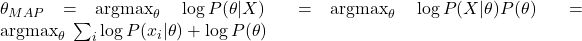Therefore, compared with MLE, MAP further incorporates the prior information. If we assume the prior distribution of the parameters to be uniform distribution, then MAP is the same as MLE.

### Case Study: Linear Regression

Linear regression is the basic model for regression analysis; its simplicity allows us to apply analytical methods. We often define the true regression value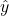following the Gaussian distribution: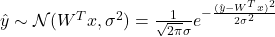where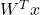is the predicted value from linear regression. We can perform both MLE and MAP analytically.

#### MLE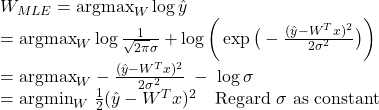We can see that if we regard the variance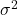as constant, then linear regression is equivalent to doing MLE on the Gaussian target.

#### MAP

As we already know, MAP has an additional prior than MLE. We assume the prior distribution P(W) as Gaussian distributionas well: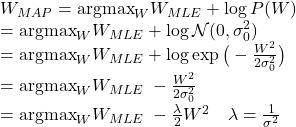We can see that under the Gaussian prior, MAP is equivalent to the linear regression with L2/ridge regularization.

References:

https://towardsdatascience.com/probabilistic-interpretation-of-linear-regression-clearly-explained-d3b9ba26823b#:~:text=Linear%20regression%20makes%202%20assumptions,value%20plus%20an%20error%20term.

https://bjlkeng.github.io/posts/a-probabilistic-view-of-regression/

https://brunomaga.github.io/Bayesian-Linear-Regression

https://medium.com/@luckecianomelo/the-ultimate-guide-for-linear-regression-theory-918fe1acb380

https://towardsdatascience.com/mle-vs-map-a989f423ae5c